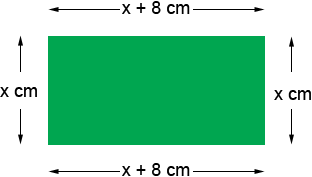Subject: Algebra/dimensions Name: Ben Who are you: Student A rectangle's length is 8 cm. greater than its width. Its perimeter is 128 cm. Find its dimensions. Hi Ben, We have two solutions for you. Did you draw a diagram?In my diagram the length of the short side is x cm so the length of the long side is x + 8 cm. Hence the perimeter is x + 8 + x + x + 8 + x cm But you know the perimeter is 128 cm. What is x? Penny and Hi Ben. The way I would solve this is to first give variable names to the two things we want to find: the length L and the width W. The perimeter is 128 so: 128 = L + W + L + W And the length is 8 more than the width so: L = W + 8 That last line means that wherever you see L, you can substitute "W + 8". So let's do that in the first equation: 128 = (W + 8) + W + (W + 8) + W Can you solve it now? Stephen La Rocque>